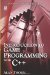# 16.4 Transformation

## 16.4 Transformation

Besides loading and painting surfaces to the display using CL_Surface, there is a great deal more power offered by this class. Specifically, there are a number of methods for applying various transformations to a surface. That is, methods to rotate, scale, and change color information. These are considered in the following subsections.

### 16.4.1 Scale

Each surface has a scale; or, to be more precise, each dimension of the surface has a scale. So, there is a scale for the x-axis and a scale for the y-axis. Mathematically, scale refers to the ratio between the size of the original image and the size at which it's painted to the display. An image 100 pixels wide that is to be painted 200 pixels wide is therefore scaled twice as large as the original; the same surface painted at only 50 pixels wide would be half the size of the original. Using a destination rectangle, we can automatically stretch an image of any size to fit inside a specified region. However, CL_Surface also offers a method to set the scale of the surface no matter which method is used to draw it. This method is set_scale. It takes two float arguments, referring to the scale of each axis. 1.0 is the default scale and represents the image's original size. A value of 0.5 will therefore represent half the original size, while 2.0 is twice the size. Consider the following code:

`      Surface.set_scale(1.0, 1.0); `

### 16.4.2 Rotation

Rotation refers to the turning of a surface about an axis. One complete revolution is 360 degrees, a half turn is therefore 180, and a quarter turn is 90 degrees. A surface can be rotated in one of two ways: set_angle or rotate. The set_angle method directly sets the angle the surface is to be rotated by; rotate is cumulative and adds a specified angle of rotation to the current angle applied to the surface. The rotation or angle of a surface can be set as follows:

`      Surface.set_angle(45); `

### 16.4.3 Color

The color of the surface can also be set. To do this, call the method set_color. This is designed to set the overall color of the surface and blend the image onto it. The set_color method accepts as an argument a CL_Color class, which can be filled in simply. CL_Color defines a color in ClanLib. It takes the form of four numbers, representing the RGBA components of a color (Red, Green, Blue, and Alpha). Each of these values can range from 0 to 255, with 0 being black and 255 being full intensity. This means if the R is 255, then red is on at full intensity, but if it is 0, it will be black. Similarly, if G is 255, it will be full green, and if blue is 255, it will be full blue. The RGB structure represents a color that is a mix of all these intensities. So, if all RGB components were 0, the final color would be black. If all were 255, then the final color would be white. The color of a surface can be set as follows:

`      Surface.set_color(r,g,b); `
 Note If the alpha component is not specified in a call to set_color, then it will default to a value of 1.0, meaning transparency is disabled.

### 16.4.4 Alpha

The alpha transparency of a surface can be set individually, without affecting color, using the set_alpha method. This method accepts any percentage value from 0 to 1, with 0% being transparent and 1% being opaque.

`      Surface.set_alpha(0.5); `Introduction to Game Programming with C++ (Wordware Game Developers Library)
ISBN: 1598220322
EAN: 2147483647
Year: 2007
Pages: 225
Authors: Alan Thorn

Similar book on Amazon

flylib.com © 2008-2017.
If you may any questions please contact us: flylib@qtcs.net